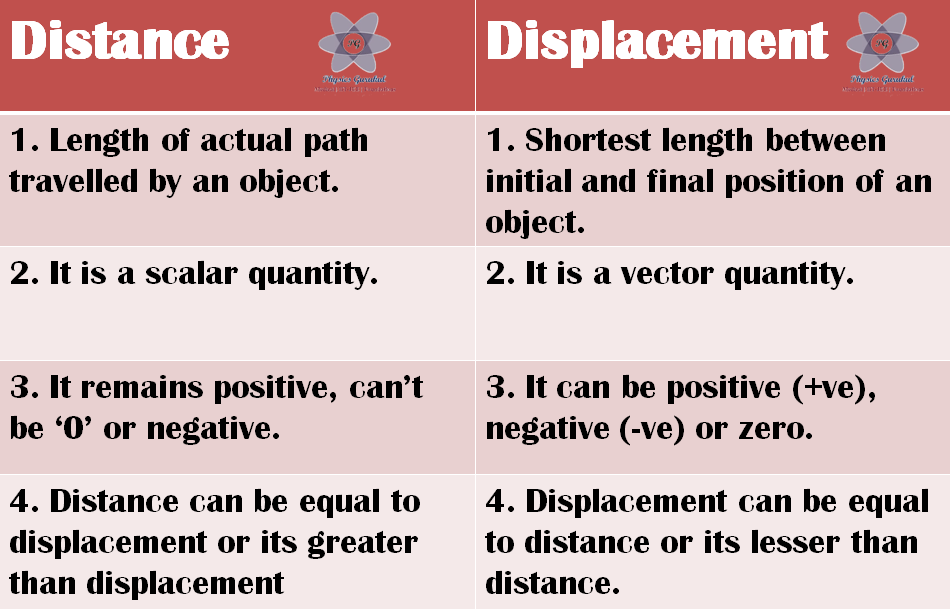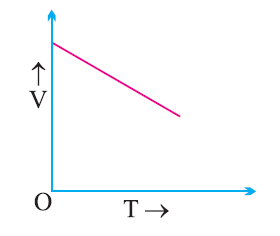# Study Notes for Class 9 Science Chapter 8 Motion

Home » CBSE Class 9 Science » Study Notes for Class 9 Science » Study Notes for Class 9 Science Chapter 8 Motion

Study Notes for Class 9 Science Chapter 8 Motion

### Topics to be covered:

• What is motion?
• Describing motion of a particle
• Position, path length and displacement
• Speed and Velocity
• Rate of change of velocity
• Distance-time graph
• Velocity-time graph
• Equations of Motion
• Uniform Circular Motion

### What is motion?

State of Rest: An object is said to be in the state of rest if it does not change its position with respect to time and its surroundings.

State of Motion: An object is said to be in the state of motion if it changes its position with respect to time and its surroundings.

Note: Rest & Motion are relative terms.

Motion can be of different types depending upon the type of path by which the object is going through.
(i) Circulatory motion/Circular motion – In a circular path.

(ii) Linear motion – In a straight line path.

(iii) Oscillatory/Vibratory motion – To and fro path with respect to origin.

Scalar quantity : Physical quantities having magnitude but no direction are called scalars. e.g., distance, speed.

Vector quantity : Physical quantities having both magnitude and direction are called vectors. e.g., displacement, velocity.

### Distance (or Path length) & Displacement:

Path length (or distance) : The actual path or length travelled by a object during its journey from its initial position to its final position is called the distance.

Note:

(i) Distance is a scalar quantity which requires only magnitude but no direction.

Example, Manju travelled 65 km.

(ii) Distance is measured by odometer in vehicles.

Displacement: It is the shortest distance between the initial and final position of a object.

Note:

(i) The shortest distance between two point is a straight line.

(ii) Displacement is a vector quantity which requires both magnitude and direction for its explanation.

Example, Nitin travelled 65 km south-west from Clock Tower.

(iii) Displacement can be zero (when initial point and final point of motion are same) Example, circular motion.

### Difference between Distance & Displacement:

(1) Distance is the length of actual path travelled by an object whereas displacement is the shortest length between initial and final position of an object.

(2) Distance is a scalar quantity whereas displacement is a vector quantity.

(3) Distance remains positive, it cant be zero or negative whereas displacement can be positive, negative or zero.

(4) Distance can be equal to displacement or its greater than displacement whereas displacement can be equal to the distance or its lesser than the distance. It can never be greater than displacement in any situation.

#### Solved Examples:

Example 1: A body travels in a semicircular path of radius 10 m starting its motion from point ‘A’ to point ‘B’. Calculate the distance and displacement.

Solution:

#Given,

π=3.14, R=10m

Total distance travelled by the object

distance=πR= 3.14 x 10 = 31.4 m

Total displacement of the body, D=2R = 2 x 10 = 20 m

Example 2. A body travels 4 km towards North then he turn to his right and travels another 4 km before coming to rest. Calculate (i) total distance travelled, (ii) total displacement.

### Solution:

Total distance travelled, = OA + AB= 4 + 4 = 8 km

Total displacement=OB=√(OA2+OB2)=√(42+42)=√32=5.65 km

### Speed and Velocity

Speed : The measurement of distance travelled by a body per unit time is called speed.

Speed = Distance travelled / Time taken

v= s/t

SI Unit: meter/second (m/s)

Velocity: It is the speed of a body in given direction.

Velocity = Displacement / Time

NOTE:

(1) velocity is a vector quantity. Its value changes when either its magnitude or direction changes.

(2) It can be positive (+ve), negative (-ve) or zero.

### Uniform and Non-uniform Motions

Uniform Motion :
When a body travels equal distance in equal interval of time, then the motion is said to be uniform motion.

Non-uniform Motion :
In this type of motion, the body will travel unequal distances in equal intervals of time.

Continuous increase in the slope of curve indicates accelerated motion. The speed of the body is increasing with time.

Continuous decrease in the slope of curve indicates decelerated motion. The speed of the body is decreasing with time.

Non-uniform motion is of two types :

(i) Accelerated Motion : When speed of a body increases with time.

The slope of v-t graph gives acceleration. The slope of the above graph is constant. so the above graph is representing a uniformly accelerated motion.

(ii) De-accelerated Motion : When speed or motion of a body decreases with time.

###NOTE:

• If a body is executing uniform motion, then there will be a constant speed.
• If a body is travelling with non-uniform motion, then the speed will not remain uniform but have different values throughout the motion of such body.For non-uniform motion, average speed will describe one single value of speed throughout the motion.

For non-uniform motion, average speed will describe one single value of speed throughout the motion.

Average speed = Total distance travelled / Total time taken

NOTE:

(I) For non-uniform motion in a given line, average velocity will be calculated in the same way as done in average speed.

Average velocity = Total displacement/Total time

(II) For uniformly changing velocity, the average velocity can be calculated as follows :

Average velocity = (Initial velocity + Final velocity)/2

Vaverage = (u + v)/2

where, u = initial velocity, v = final velocity

### Conversion factor:

1 km/hr = 1000 m / 3600 s =5/18 m/s

Note:

To convert from km/hr to m/s → multiply by 5/18

To convert from m/s to km/hr → multiple by 18/5

Examples:

(1) 72 km/hr = 72 x 5/18 = 20 m/s

(2) 54 km/hr = 54 x 5/18 = 15 m/s

(3) 50 m/s = 50 x 18/5 = 180 km/hr

### Solved Examples:

Example : What will be the speed of body in m/s and km/hr if it travels 40 km in 5 hrs ?

Solution :

Distance (s) = 40 km

Time (t) = 5 hrs.

Speed = Total distance / Total time = 40 km/ 5 hrs. = 8 km/hr. (in km/hr)

Speed in m/s:

8 km/hr = 8 x 5/18 = 40/18 = 2.22 m/s

Acceleration : Acceleration is seen in non-uniform motion and it can be defined as the rate of change of velocity with time.

Acceleration = Change in velocity / Time

a= (v-u)/t

where, v = final velocity, u = initial velocity
If v > u, then ‘a’ will be positive (+ve).

Retardation/Deceleration : Deceleration is seen in non-uniform motion during decrease in velocity with time. It has same definition as acceleration.

Deceleration = Change in velocity / Change in time

a’=(v-u)/t

Here v < u, ‘a’ = negative (-ve).

### Solved Examples:

Example 1 : A car speed increases from 40 km/hr to 60 km/hr in 5 sec. Calculate the acceleration of car.

Solution :

Given,

u = 40 km/hr = 40 x 5/18 = 11.11 m/s

v = 60 km/he = 60 x 5/18 = 16.66 m/s

a = ?

We know that,

a=(v-u)/t = (16.66-11.11)/5 = 5.55/5 = 1.11 m/s2

Example 2. A car travelling with a speed of 20 km/hr comes into rest in 0.5 hrs. What will be the value of its retardation ?

Solution :

Given,

v = 0 km/hr

u = 20 km/hr

t = 0.5 hrs.

Retardation, -a = ?

We have,

a =(v-u)/t = (0-20)/0.5 = -40 km/hr2

Therefore, retardation = 40 km/hr2

### Equation of Motion (For Uniformly Accelerated Motion)

(i) First Equation:

v = u + at

Final velocity = Initial velocity + Acceleration × Time

Graphical Derivation :

Suppose a body has initial velocity ‘u’ (i.e., velocity at time t = 0 sec.) at point ‘A’ and this velocity changes to ‘v’ at point ‘B’ in ‘t’ secs. i.e., final velocity will be ‘v’.

For such a body there will be an acceleration.

(ii) Second Equation:

Distance travelled by object

(iii) Third Equation:

### Uniform Circular Motion:

If a body is moving in a circular path with uniform speed, then it is said to be executing uniform circular motion. In such a motion the speed may be same throughout the motion but its velocity (which is tangential) is different at each and every point of its motion. Thus, uniform circular motion is an accelerated motion.

In order to equip the students with the latest methodology of testing and evaluation in competitive exam segment in India Physics Gurukul has introduced successrouter.com for Online Testing and Assessment. Try our free tests for JEE and NEET Today!

PowerPoint Presentations (PPTs) for Class 7 to 12